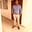RELATED TAGS

pandas
python

# How to obtain the modal value over specified axes of a dataframeOnyejiaku Theophilus Chidalu

## Overview

The mode() function in pandas is used to obtain the modal value of the elements along a specified axis of the given dataframe.

Mathematically, the mode can be defined as the value of a dataset with the highest frequency or the highest number of occurrences.

### Syntax

The mode() function takes the syntax below:

DataFrame.mode(axis=NoDefault.no_default, skipna=True, numeric_only=None, **kwargs)
Syntax for the mean() function in pandas

### Parameter value

The mode() function takes the following optional parameter values:

• axis: This represents the name for the row (designated as 0 or index) or the column (designated as 1 or columns) axis.
• numeric_only: This takes a boolean value indicating if to include only float, int, and boolean columns.
• dropna: This takes a boolean value indicating if NaN or NaT values are not to be considered.

### Return value

The mode() function returns a dataframe with modal values across the specified axis.

### Code example

Let’s look at the code below:

# A code to illustrate the mode() function in Pandas

# importing the pandas library
import pandas as pd

# creating a dataframe
df = pd.DataFrame([[1,2,3,4,5],
[1,7,5,9,0.5],
[3,11,13,14,12]],
columns=list('ABCDE'))
# printing the dataframe
print(df)

# obtaining the modal value vertically across rows
print(df.mode())

# obtaining the modal value horizontally over columns
print(df.mode(axis="columns"))
Implementing the mode() function

### Code explanation

• Line 4: We import the pandas library.
• Lines 7 to 10: We create a dataframe called df.
• Line 12: We print the dataframe, df.
• Line 15: We use the mode() function to obtain the modal values running downwards across the rows (axis 0). We print the result to the console.
• Line 18: We use the mode() function to obtain the modal values running horizontally across columns (axis 1). We print the result to the console.

RELATED TAGS

pandas
python

CONTRIBUTOROnyejiaku Theophilus Chidalu
RELATED COURSES

View all Courses

Keep Exploring

Learn in-demand tech skills in half the time